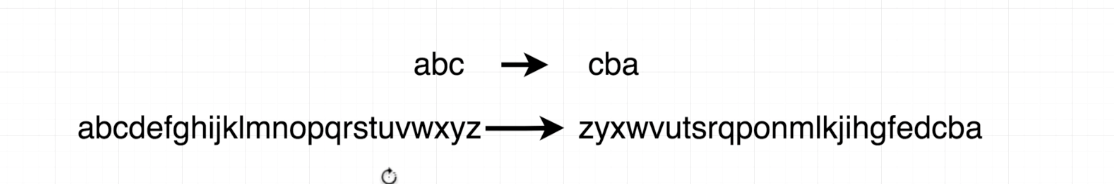Runtime Complexity and Big(O)

Whenever you tackle a problem in code, there is a good chance that the solution you come up with will not be identical to solutions arrived at by other developers. Maybe one developer likes building character maps, while another likes bubble sorting. Depending on what you’re working on this MAY (notice the caps there) not be a big deal. However, in many cases you DO need to pay attention to exactly which solution you choose. Why? Isn’t the point to just solve a problem, no matter how it’s done? Obviously, since I’m asking the question, the answer is clearly no…you are not just looking at solving a problem. You are looking at how to BEST solve the problem.

So how do developers determine what the “best” solution is? Enter something called Runtime Complexity, which is used to describe the performance of an algorithm. Whenever you are determining how to solve a coding problem, you are looking to choose the MOST EFFICIENT solution in terms of performance. You need to always be asking yourself: how much more processing power will be needed to run my chosen algorithm or solution if the number of inputs into that algorithm is increased?

A good example of how to think of Runtime complexity can be found in a simple algorithm where you are required to reverse a string.There is a 1:1 relationship here between input and amount of work

For each additional element in the string, you will have to iterate one more time through the loop…so ONE additional input requires ONE additional step. This is why we say there is a 1:1 relationship between the amount of work required and the input set. Therefore, this is an example of Linear Runtime Complexity, which is one of the most common types of algorithmic complexities.

Let’s look at another example that illustrates what happens at the other end of the complexity spectrum, with something that takes more processing power. I’ve seen this called the “steps” problem, where you are given a positive integer, N. Your job is to write a function that accepts N and console logs a step shape with N levels, using the ‘#’ character…there should be spaces on the right hand side for all steps having less than N number of ‘#’ characters.

Here is the iterative solution to that algorithm:

Now, what kind of runtime complexity does this illustrate? Well, what happens each time we increase the number of “steps” we pass into our function?

In this solution, we see that there are two for loops, one nested inside of the other. As we started to increase the value we input into our algorithm, we had to do many more things each time n was increased by 1. This is a good example of Quadratic Runtime Complexity, or .

So these two examples (that are found essentially at differing ends of the complexity spectrum) can really help our brains establish some kind of framework around which we can construct our understanding of runtime complexity. Unfortunately, there is no magic formula that you can be given that 100% of the time always identifies the correct runtime complexity nor is there any hard and fast rule as to exactly how this stuff is done. Exposure to different algorithms and practicing the best solutions are really the only ways to get a full grasp of the processing power required. That being said, it helps to know some common runtimes and examples of those runtimes.

Some Common Runtimes and Examples…and, oh yeah, Big(O) notation.

Ok, now on to some common types of Runtime complexities and examples.

Constant. No matter what our input is, it will always take the exact same amount of time. This is the “holy grail” of code but pretty much never happens. You could think of an example of this as having to always print out just the first element of a collection, no matter how long the collection is. In Big(O) notation this is represented as O(1).

Logarithmic. This type of complexity is found if we double the input but the amount of work we do does not exactly double. An example of this would be searching through sorted data. In Big(O) notation this is represented as O(log(n)).

Linear. This is the most common type of complexity. You will see this if you are iterating through a single fixed collection of data; for example, if you are using a for loop that runs from zero to array.length. In Big(O) notation this is represented as O(n). In addition, if you are iterating through two different collections with separate for loops you can express this as: O(n + m).

Quasilinear. This type of complexity is seen if, increasing input by 1, the amount of work increases by 1 plus some small amount (so not linear but not quite double). This is the complexity exhibited by many different sorting algorithms. In Big(O) notation this is represented as O(n*log(n)).

Quadratic. You will see this complexity when every element in a collection has to be compared to every other element. In other words, as you increase input, it takes dramatically more time to complete the algorithm. Remember our ‘steps’ algorithm solution…this type of complexity is seen when you have two nested for loops iterating over the same collection. In Big(O) notation, this is represented as O(n²).

Exponential. Finally, this type of complexity is seen if, for every single element added, the processing power DOUBLES. This is obviously a significant increase in processing power and you REALLY do not want this. In Big(O) notation, this is represented as O(2^n).

As I mentioned above, the more you get used to thinking about the runtime complexity of bits of code, the quicker you will get at identifying the most efficient solution to employ. It definitely takes some getting used to at first, but with time you’ll find yourself automatically asking “which of these potential solutions has the better runtime?”

Happy coding!